Changing the coordinate system

INTRODUCTION

In this part of the course, we introduce the basics of 2D transformations, a powerful tool that will make things easier as soon as you have to:

• Draw complex shapes at given positions, with given orientations and sizes,
• Draw shapes relative to one another.

Let’s start with some simple examples before looking at how we use 2D transforms.

LET’S DRAW THREE RECTANGLES!

If we draw three rectangles of size 100×200 in a 400×400 canvas, one at (0, 0) and another at (150, 0), and a third at (300, 0), here is the result and the corresponding code: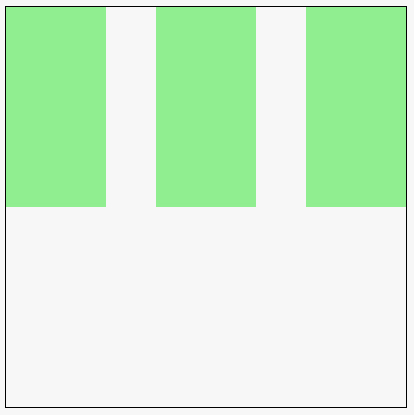JavaScript code extract (see the online example at JS Bin for the complete running code)

1. function drawSomething() {
2.      ctx.fillStyle=‘lightgreen’;
3.
4.      ctx.fillRect(0,0,100,200);
5.      ctx.fillRect(150,0,100,200);
6.      ctx.fillRect(300,0,100,200);
7. }

LET’S MODIFY THE CODE SO THAT WE CAN DRAW THESE RECTANGLES AT ANY X AND Y POSITION

What if we wanted to draw these 3 rectangles at another position, as a group? We would like to draw all of them a little closer to the bottom, for example… Let’s add some parameters to the function:  the X and Y position of the rectangles.

The full JavaScript code is (see online running example):

1. var canvas, ctx;
2. function init() {
3.     // This function is called after the page is loaded
4.     // 1 – Get the canvas
5.     canvas = document.getElementById(‘myCanvas’);
6.     // 2 – Get the context
7.     ctx=canvas.getContext(‘2d’);
8.     // 3 – we can draw
9.     drawSomething(0, 100);
10. }
11. function drawSomething(x, y) {
12.     // draw 3 rectangles
13.     ctx.fillStyle=‘lightgreen’;
14.     ctx.fillRect(x,y,100,200);
15.     ctx.fillRect(x+150,y,100,200);
16.     ctx.fillRect(x+300,y,100,200);
17. }

At line 10, we called the drawSomething(…) function with 0 and 100 as parameters, meaning “please add an offset of 0 in X and 100 in Y directions to what is drawn by the function…

If you look at the code of the modified function, you will see that each call to fillRect(…) uses the x and y parameters instead of hard coded values. In this way, if we call it with parameters (0, 100), then all rectangles will be drawn 100 pixels to the bottom (offset in y). Here is the result: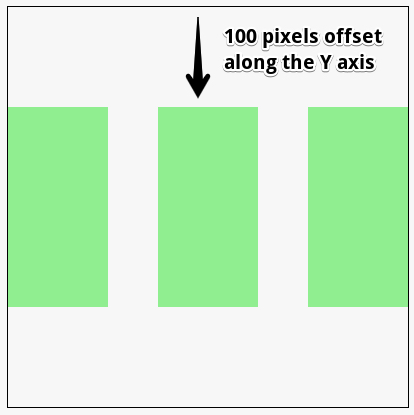OK, NOW LET’S DRAW A SMALL MONSTER’S HEAD WITH RECTANGLES

Now we can start having some fun… let’s draw a monster’s head using only rectangles (online version):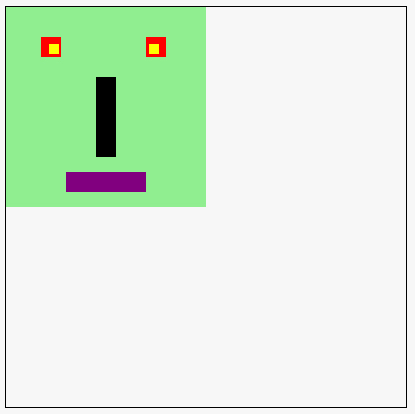An extract of the JavaScript source code is:

1. function drawMonster(x, y) {
3.    ctx.fillStyle=‘lightgreen’;
4.    ctx.fillRect(x,y,200,200);
5.    // eyes
6.    ctx.fillStyle=‘red’;
7.    ctx.fillRect(x+35,y+30,20,20);
8.    ctx.fillRect(x+140,y+30,20,20);
9.    // interior of eye
10.    ctx.fillStyle=‘yellow’;
11.    ctx.fillRect(x+43,y+37,10,10);
12.    ctx.fillRect(x+143,y+37,10,10);
13.    // Nose
14.    ctx.fillStyle=‘black’;
15.    ctx.fillRect(x+90,y+70,20,80);
16.    // Mouth
17.    ctx.fillStyle=‘purple’;
18.    ctx.fillRect(x+60,y+165,80,20);
19. }

As you can see, the code uses the same technique, becomes less and less readable. The Xs and Ys at the beginning of each call makes understanding the code harder, etc.

However, there is a way to simplify this => 2D geometric transformations!

GEOMETRIC TRANSFORMATIONS: CHANGING THE COORDINATE SYSTEM

The idea behind 2D transformations is that instead of modifying all the coordinates passed as parameters to each call to drawing methods like fillRect(…), we will keep all the drawing code “as is”. For example, if the monster of our previous example was drawn at (0, 0), we could just translate (or rotate, or scale) the original coordinate system.

Let’s take a piece of code that draws something corresponding to the original coordinate system, located at the top left corner of the canvas:

1. function drawMonster(x, y) {
3.    ctx.fillStyle=‘lightgreen’;
4.    ctx.fillRect(0,0,200,200);
5.    // eyes
6.    ctx.fillStyle=‘red’;
7.    ctx.fillRect(35,30,20,20);
8.    ctx.fillRect(140,30,20,20);
9.    // interior of eye
10.    ctx.fillStyle=‘yellow’;
11.    ctx.fillRect(43,37,10,10);
12.    ctx.fillRect(143,37,10,10);
13.    // Nose
14.    ctx.fillStyle=‘black’;
15.    ctx.fillRect(90,70,20,80);
16.    // Mouth
17.    ctx.fillStyle=‘purple’;
18.    ctx.fillRect(60,165,80,20);
19.    // coordinate system at (0, 0)
20.    drawArrow(ctx, 0, 0, 100, 0, 10, ‘red’);
21.    drawArrow(ctx, 0, 0, 0, 100, 10, ‘red’);
22. }

This code is the just the same as in the previous example except that we removed all Xs and Yx in the code. We also added at the end (lines 25-26) two lines of code that draw the coordinate system. The drawArrow(startX, startY, endX, endY, width, color) function is a utility function that we will present later. You can see it in the source code of the complete online example on JS Bin: look in the JavaScript tab.

Note that the X and Y parameters are useless for now…

Result: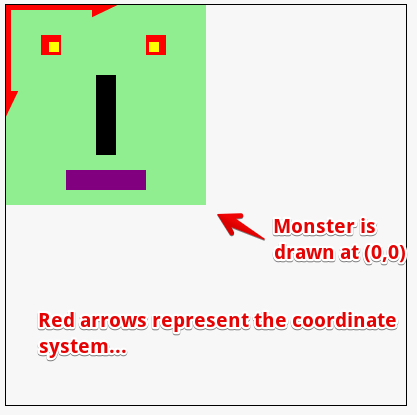Translation using ctx.translate(offsetX, offsetY)

Now, instead of simply calling drawMonster(0, 0), we will call first ctx.translate(100, 100), and look at the result (online code: http://jsbin.com/yuhamu/2/edit)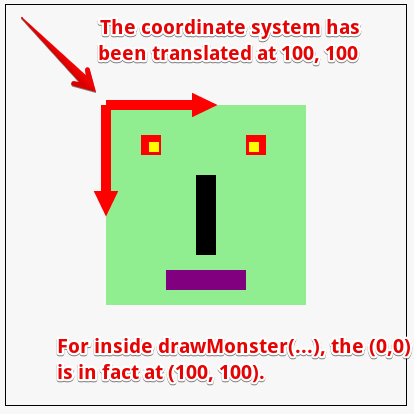JavaScript code extract:

1. ctx.translate(100, 100);
2. drawMonster(0, 0);

Line 1 changes the position of the coordinate system, line 2 draws a monster in the new translated coordinate system. All subsequent calls to drawing methods will be affected and will work in this new system too.

OTHER TRANSFORMATIONS: ROTATE, SCALE

There are other transformations available:

• ctx.rotate(angle), with angle in radians. Note that the order of transformations is important: usually we translate, then rotate, then scale… If you change this order, you need to know what you are doing…
• ctx.scale (sx, sy), where scale(1, 1) corresponds to “no zoom”, scale(2, 2) corresponds to “zooming 2x” and scale(0.5, 0.5) corresponds to zooming out to see the drawings half as big as before. If you do not use the same values for sx and sy, you do “asymmetric scaling”, you can distort a shape horizontally or vertically. Try changing the values in the source code of the next online examples.

Here is the previous example, but this time we translated the coordinate system, then rotated it with an angle equal to PI/4 , then we scaled it so that units are half as big (see the example online):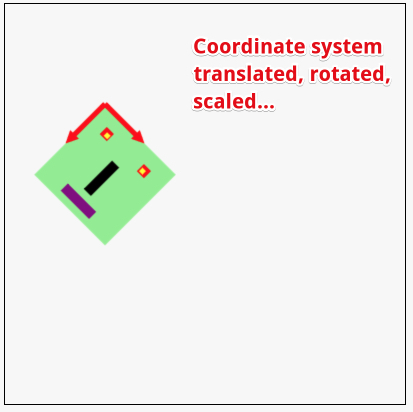And here is the code of the transformations we used, followed by the call to the function that draws the monster:

1. ctx.translate(100, 100);
2. ctx.rotate(Math.PI/4);
3. ctx.scale(0.5, 0.5);
4.
5. drawMonster(0, 0);

Line 1 does: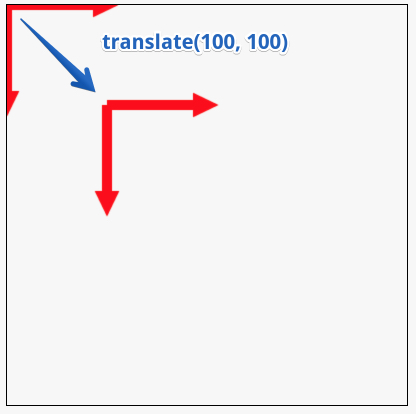Line 2 does: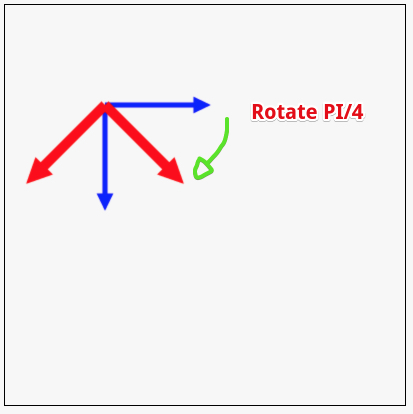Line 3 does: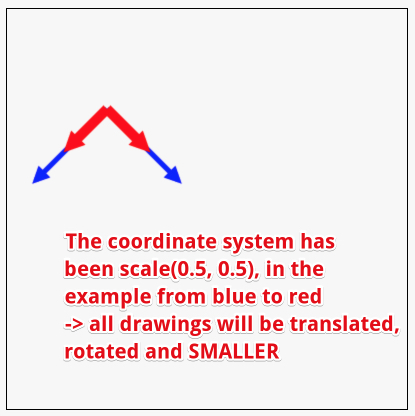Line 4 draws the monster in this new translated, rotated, scaled coordinate system:BEWARE: ALL DRAWINGS TO COME WILL BE IN THAT MODIFIED COORDINATE SYSTEM!

If we draw two shapes at two different positions, they will be relative to this new coordinate system.

For example, this code (online at http://jsbin.com/yuhamu/4/edit):

1. ctx.translate(100, 100);
2. ctx.rotate(Math.PI/4);
3. ctx.scale(0.5, 0.5);
4. // Draw the monster at (0, 0)
5. drawMonster(0, 0);
6. // Draw a filled rectagle at (250, 0)
7. ctx.fillRect(250, 0, 100, 100);

… gives this result: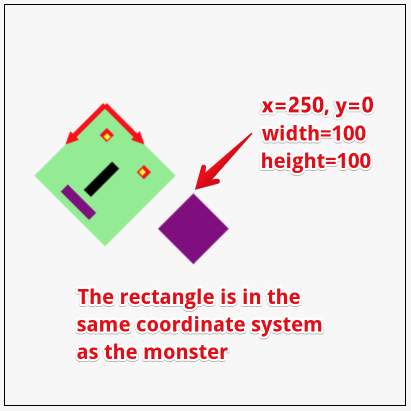HOW CAN WE RESET THE COORDINATE SYSTEM, HOW CAN WE GO BACK TO THE PREVIOUS ONE?

Aha, this is a very interesting question… we will give the answer very soon in the section on saving/restoring the context 🙂